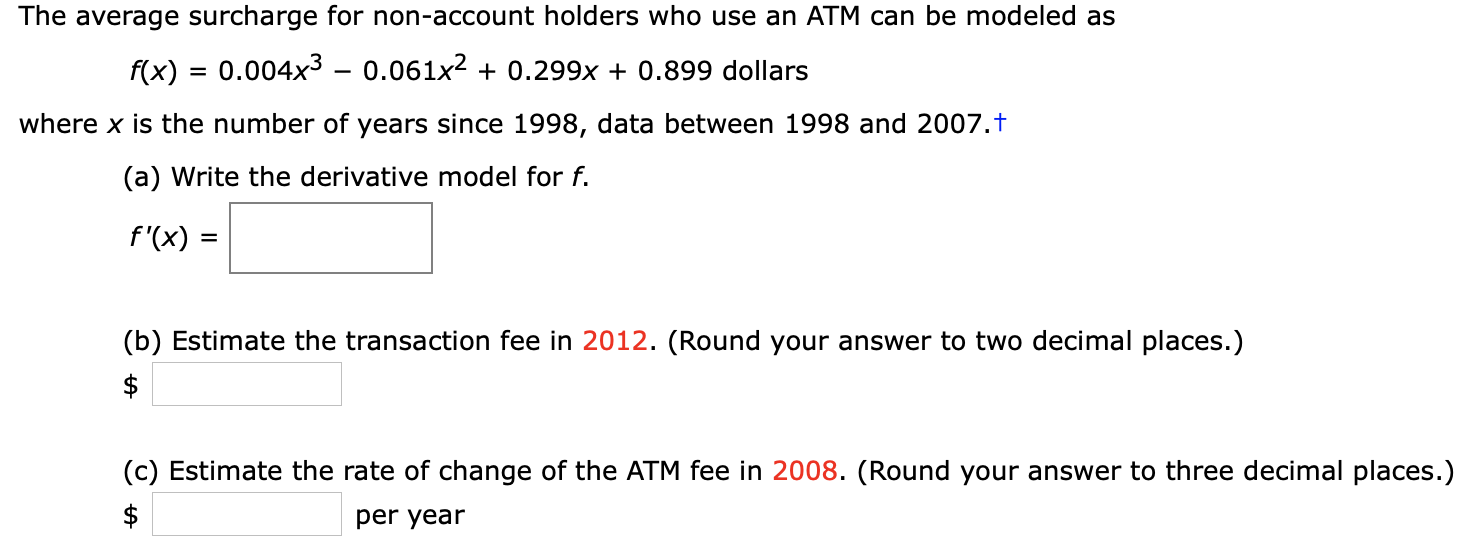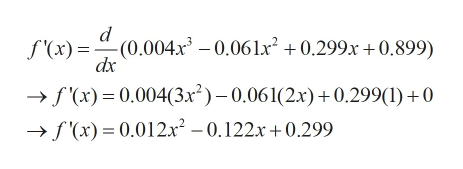The average surcharge for non-account holders who use an ATM can be modeled as0.004x3 - 0.061x20.299x + 0.899 dollarsf(x)where x is the number of years since 1998, data between 1998 and 2007.t(a) Write the derivative model for f.f'(x)(b) Estimate the transaction fee in 2012. (Round your answer to two decimal places.)\$(c) Estimate the rate of change of the ATM fee in 2008. (Round your answer to three decimal places.)\$per year+A

Questionhelp_outlineImage TranscriptioncloseThe average surcharge for non-account holders who use an ATM can be modeled as 0.004x3 - 0.061x2 0.299x + 0.899 dollars f(x) where x is the number of years since 1998, data between 1998 and 2007.t (a) Write the derivative model for f. f'(x) (b) Estimate the transaction fee in 2012. (Round your answer to two decimal places.) \$ (c) Estimate the rate of change of the ATM fee in 2008. (Round your answer to three decimal places.) \$ per year +A fullscreen
Step 1

Given the average surcharge is

Step 2

Differentiate both the sides with respect to xhelp_outlineImage Transcriptionclosed -(0.004x3 - 0.06lx2 +0.299x 0.899) dx f(x) = f(x) 0.004(3x2)-0.061(2.x)+0.299(1)0 -»f'(x) 0.012x2 - 0.122x+0.299 fullscreen
Step 3

Plug x=14 in f(x) to get the transaction fee in 2012 since the differen...

Want to see the full answer?

See Solution

Want to see this answer and more?

Our solutions are written by experts, many with advanced degrees, and available 24/7

See Solution
Tagged in

Functions